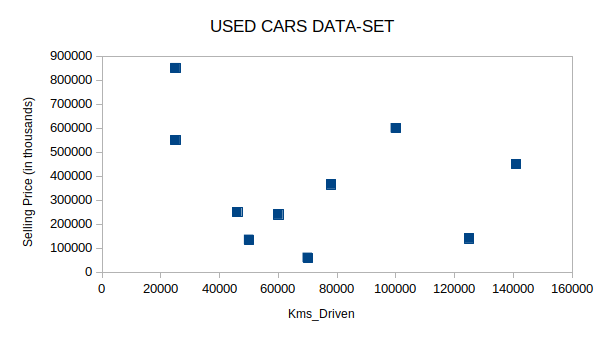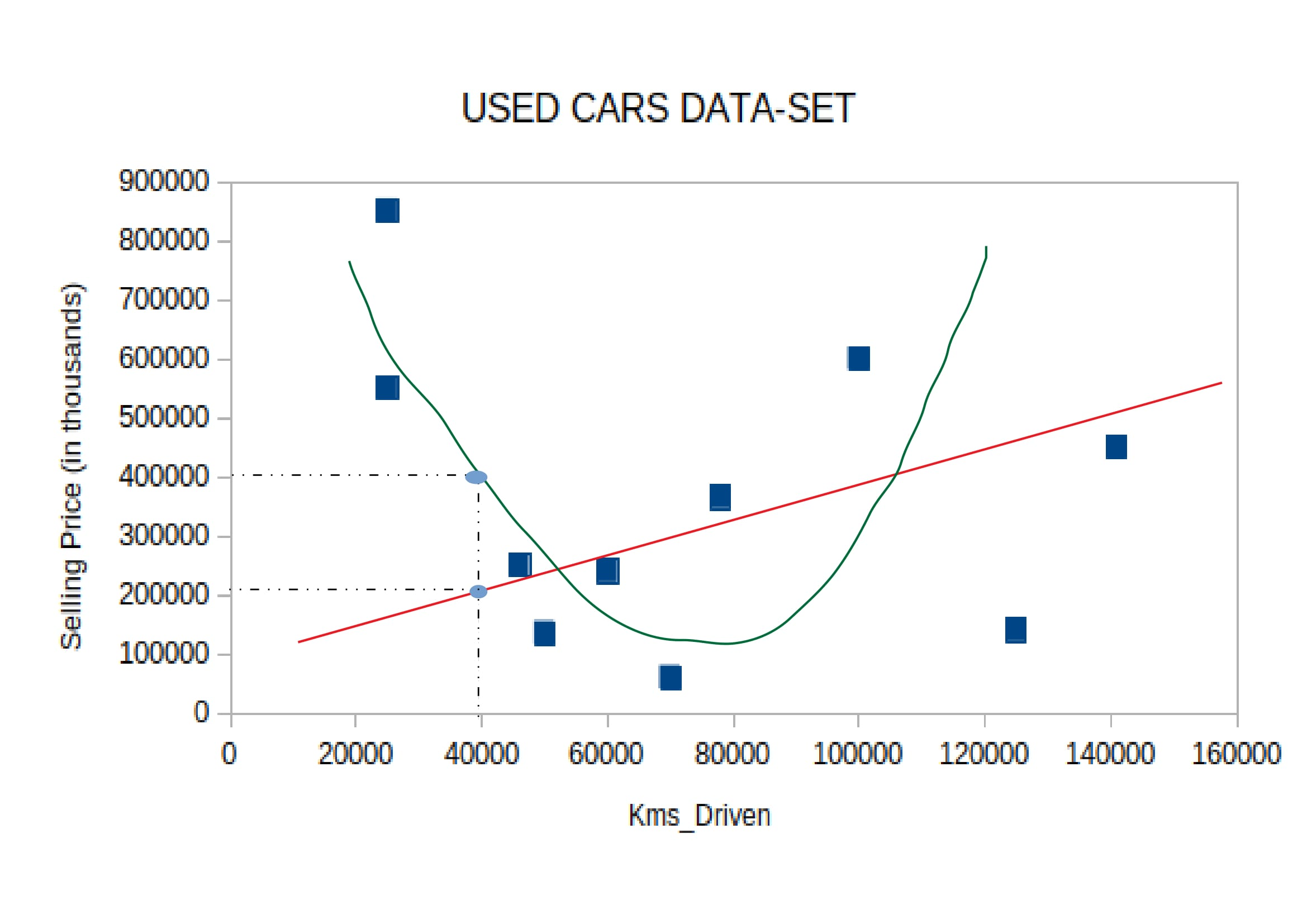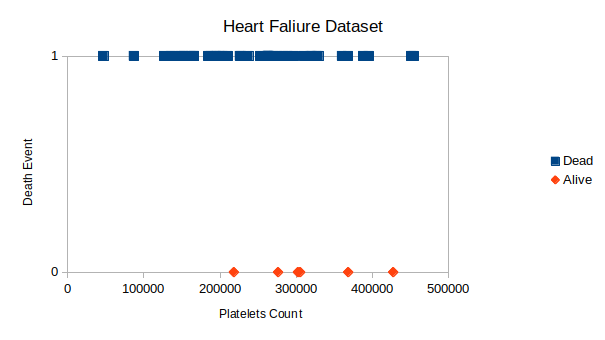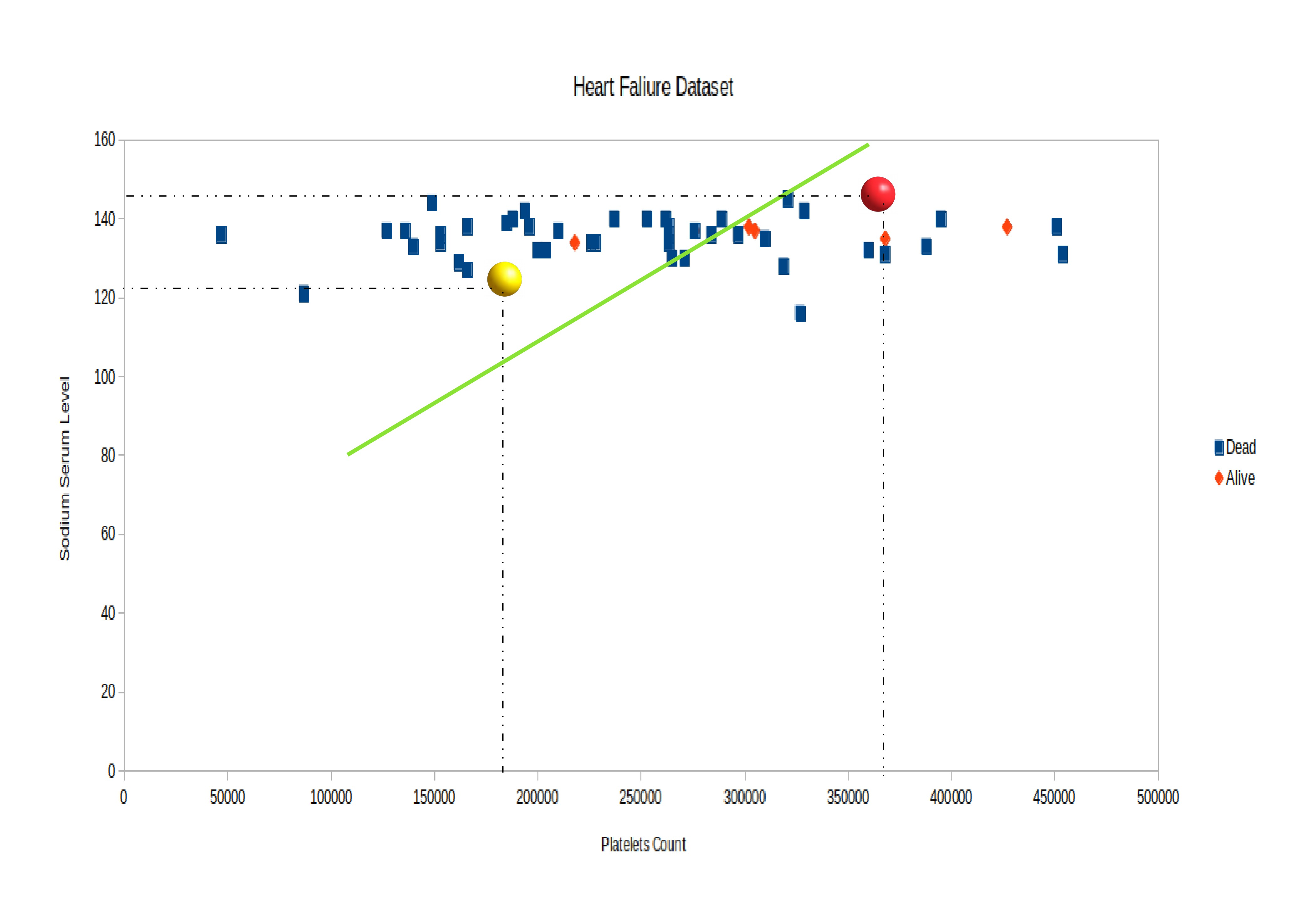# SUPERVISED LEARNING

Posted by Ashutosh on February 12, 2021

In Supervised Learning, we know the possible outcome. We adequately provide historical data to the standard algorithm and get the desired result. We trained the model on a labeled dataset. The labeled dataset means we have both input and output parameters.

Image Classification represents a classic example of Supervised Learning. The algorithm trained enough to identify the image accurately. When the user provides the image to the system, based on its data, it will recognize the type of image.

Supervised Learning Algorithms

• Linear Regression
• Logistic Regression
• Nearest Neighbor
• Decision Trees
• Support Vector Machine (SVM)
• Random Forest
• Neural Networks (most of them)

Now let's discuss supervised learning in much detail based on its categorization. But before we establish a formal definition of what supervised learning is, let us understand it with the help of examples.

## Example-1

Say, there is data available on used car prices. Since the data is labeled (available output value corresponding to each set of input values) we need to develop a learning model to train on this data to know the right answers (correct output value) for the new set of input. The aim is to predict the selling price of a used car based on the number of kilometers it has completed.The X-axis represents the number of kilometers the car has been driven and the y-axis represents the corresponding selling price of the vehicle. We need a learning model that predicts the correct price of the vehicle for a new input after training on this data. The value to be predicted is a continuous-valued output. Such types of problems is referred as Regression Problems.

Now, suppose we make a line fit into this data-set corresponding to our training model to make predictions or we can fit in a curve(non-linear functional form) to increase the output proficiency. This choice is statistical and shall be discussed later in the course.As we see in the above figure, a red straight line and a green quadratic curve fit into the data-set in such a way that it covers most of the data points. We can predict the response of our model on a new set of inputs based on these curves. Suppose, we need to know the selling price of a used car that had run 40,000 km. According to the first learning model i.e. linear, the selling price of the car will be Rs. 200,000 while according to the parabolic curve, the selling price of the car will be Rs. 400,000. Which of them is correct? It depends on the calculated efficiency of our learning model on the training data.

## Example-2

Now, if we have the data in which the output value is finitely categorized or is the set of discrete values then such type of problems are referred to as Classification Problems. They are most prominently dealt with, in the real world.

The learning problems in which, based on some attributes or input features, if we need to predict whether a patient suffering from Covid-19 can have a lung collapse or not in the future or if we need to predict the digits written on the driving plate of a car or to enable a machine to ratify the distinction between an aeroplane and a helicopter. Each of the above is a typical classification problem as the output being predicted contains a discrete set of values.

The image below shows the data of the patients who had heart failure depending upon the platelet count. There can be many attributes that suggest the reason for the heart failure but for the sake of understanding easily on a 2-D surface we are focusing on a singular attribute for now i.e. platelets count.

You can access the Heart Failure Dataset(with one attribute) from below:-

Heart Failure DatasetBlue Dots shows the patients who had a heart failure corresponding to the value of platelets count on the X-axis whereas orange dots suggests people who didn't suffer heart failure. This is a classification problem because we have only two classes of values as output labels i.e. Heart Failure (1) and No Heart Failure (0).

Suppose, a second attribute that heart failure depends on is sodium serum level. Now the output value depends on two input attributes i.e. platelets count and sodium serum levels. The image below shows a green line drawn through our data. The classification learning model enables us to draw a curve (here it is a line segment) that will act as a boundary between the two classes of output labels. Any new data point that lies left of the line is predicted as a heart failure (1) and any data point to the right as no heart failure (0). Yellow and Red balls are the two new data points that are predicted as heart failure and no heart failure respectively.Supervised Learning - Categorization

Supervised Learning is a type of machine learning in which for each observation of input attribute(s) there is an associated value of response (output) recorded that is used as a data to train the learning model.

As the name suggests supervised, it means there is a supervisor under whose guidance the process of learning undergoes. Imagine a computer as our student whom we as a teacher/supervisor wishes to teach what a boat will look like. We show it various pictures that are of the boat and also those that are not the pictures of a boat, maybe of a plane, car or even a submarine. We allow it to distinguish a boat from other objects through the initial experience of learning that it gains based on the data we provide it. Variable represents data and hence can be characterized as either Quantitative and Qualitative(or Categorical). Quantitative Variable takes on the numerical values such as weight of a person, the height of the building, income, etc. On the flip side, Qualitative Variables contains a P many discrete set of categories or classes such as the color of eye (back or brown), job Grade (A, B or C), heart disease (yes or no), etc.

As we have discussed above with the help of examples it must be quite clear to you now that supervised learning is divided into two types of problems i.e.,

1. Regression Problems - The problems in which the response to be predicted is a continuous valued output such as predicting the selling price of used cars or the prediction of housing prices. We keep the problems with a quantitative response as Regression Problems.

2. Classification Problems - The problems in which the response to be predicted is a discrete-valued output such as predictions of heart failure or predicting whether the image is of a dog or cat. Classification problems are widely implemented nowadays everywhere from google lens to its wide implementation in healthcare units. Hence, problems that involve qualitative response are referred to as Classification Problems.

## Supervised Learning - Algorithms

Now, there are numerous amounts of supervised learning algorithms available, thanks to the long years of diligent research. There are classical historical methods and also the modern ML techniques work efficiently on complex humongous datasets. Let's briefly discuss each algorithm that we will be scrutinizing in a later course.

1. Linear Regression - It is a classical supervised learning model used to predict quantitative values. It has been used in statistics for decades to draw out inferences between one or more predictors (independent variable) and the response (dependent variable). As we have seen in example one, it is a Regression Algorithm where we tend to fit a linear functional form of "f" into our dataset. It is a parametric based method for estimating f. In case the response variable depends on the single predictor variable than such type is known as Simple Linear Regression while when there are one or more predictor variables involved then such algorithm is known as Multiple Linear Regression.
2. Logistic Regression - It is also a classical supervised learning model used to predict qualitative (or categorical) values. Contradicting against its name, it is a Classification Algorithm. It uses a logistic function to predict a binary response variable (0 or 1 / 'yes' or 'no'). Though more advanced versions of these algorithms are available it serves the root for each one of them. It is also based on the parametric estimator approach. It is also known as Binary Regressor where we predict 2 classes of categorical response. There is also another version of it, known as Multi-Class Classification where we predict more than 2 classes of categorical response.
3. K nearest neighbors - It is a supervised learning algorithm that is simple, easy to implement, and can be successfully applied to both regression and classification problems. K nearest neighbor deals with the concept of assuming that similar things cling near each other. For a particular test data, we tend to find the K (some positive integer) nearest data points from our training data surrounding the test data. The distance of each data point from the test data is then computed and arranged in ascending order. Most preferably we tend to find Euclidean Distance (straight line distance) but also depending upon the problem we can consider computing other distances such as Hamming DistanceManhattan Distance, or the Cosine Distance.
4. Decision Trees - Decision Trees can also be applied to both regression and classification problems. It involves creating segments out of the predictors in the training data into simple stratified regions. Predictions for any given data is done using the mean and mode of the data points belonging to that particular region. As the segmentation of data is done in the form of a tree-like structure, hence such algorithms are known as Decision Trees methods. The tree representation is used to predict an outcome where each leaf node suggests a different class label whereas the internal nodes of the tree correspond to the various predictors in our training dataset.
5. Artificial Neural Networks - As the name suggests it has its motivation incentivize from the idea of how a human brain works. It provides series of algorithms to understand the underlying relationship between data and eventually helps in giving accurate predictions. It is not specifically a machine learning algorithm but it is a way of doing machine learning. Briefly saying, it is an architectural form of applying ML most efficiently. It works well with the complex non-linear dataset. Though most of the Neural Network algorithms are aimed for supervised learning problems, it also implements unsupervised learning. Artificial Neural Networks lays the groundwork for the implementation of Deep Learning which is the technology on which modern AI works. From apple's Siri to google's Google Lens cannot be implemented without Artificial Neural Networks. Though ANN requires a completely different series of courses we will try to make you aware of its uses and implementations in this course by implementing various ANN algorithms such as Forward Propagation, Back PropagationCNN, and RNN.
6. Support Vector Machine (SVM)Support Vector Machine is a learning method used for classification problems. It was developed back in 1990 and again it's popularity based on the fact that it works exceptionally well for non-linear datasets. It enables us to draw a non-linear decision boundary in our data set. It is considered one of the best algorithms for classification problems. It is a generalized version of Maximal Margin Classifier which was an elegant method for the prediction of qualitative values but it demands that different classes of response be separated by a linear class boundary. This restriction was ended by the introduction of SVM. It can accommodate nonlinear class boundaries. SVM works for Binary Classification where there are two classes and also for Multi-class Classification where there are more than two classes.

In the upcoming articles, we discuss all algorithms in detail.